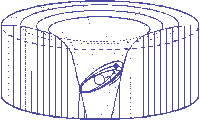Einstein's Theory of Relativity

## Classical Mechanics

by   Paul Marmet
Chapter Eleven

Internal Phenomena inside Atoms.

11.1 - Introduction.
In this chapter, we will give a physical description of the absolute changes that happen inside an hydrogen atom when it is accelerated to a high velocity. We have seen that this acceleration produces an increase of the electron mass and in the Bohr radius. We also notice from chapter three that the principle of mass-energy conservation is respected inside the hydrogen atom without having to involve any change of electric charge when the hydrogen atom is brought to high velocity. We will now show how the absolute parameters of the hydrogen atom change when it acquires kinetic energy. We present some considerations to the problem of internal potentials inside the nucleus of atoms. Finally, we will see how the nature of the interactions taking place inside nuclei can be predicted using these considerations.

11.2 - Transformations inside Fast Moving Atoms.
We have seen in equation 3.4 that when the velocity of the hydrogen atom increases, the absolute value of the Bohr radius a increases according to:

 av[rest] = gao[rest] 11.1
where ao[rest] is the Bohr radius at rest in rest units, and av[rest] is the Bohr radius at velocity v, also in rest units. The units in this chapter will always be rest units so that we will drop the index [rest]. Let us use a numerical example to illustrate some of the absolute changes taking place inside atoms accelerated to a high velocity. When an hydrogen atom moves at v = 0.866c, then g = 2. We will consider the hydrogen atom in its ground state but one can see that the transformations can be applied, in a similar way for any excited state. From equation 11.1, when v = 0.866c, we have:
 av = 2ao 11.2
We know from equation 2.23 that the electron mass increases when it moves faster:
 mv = gmo 11.3
Therefore the absolute electron mass of a hydrogen atom moving at velocity v = 0.866c becomes:
 mv = 2mo 11.4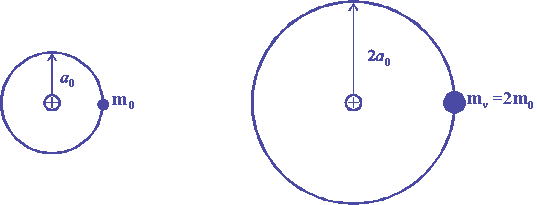Figure 11.1

Figure 11.1, illustrates the simultaneous increase of the Bohr radius and of the electron mass when g = 2. Let us examine how these results are compatible with the Bohr model, the de Broglie wavelength of particles and quantum mechanics.

11.3 - Electric Potentials.
Let us examine first the compatibility between the description given above with the laws regulating the electron and the proton in the hydrogen atom. We recall that when an atom is accelerated to a high velocity, the electric charges and the absolute electric field around those charges do not have to change in order to remain compatible with the principle of mass-energy conservation. The electric energy Eo of the electron in the electric field of the proton is: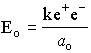11.5
where k is the Coulomb constant, e+ and e- are the electric charges of the proton and the electron and ao is the average distance between the electron and the proton which corresponds to the Bohr radius. Putting equation 11.2 in 11.5, we find that the internal electric energy Ev inside the moving hydrogen atom is: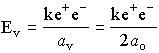11.6
Since the compatibility between observations and mass-energy conservation has been obtained without modifying the electric charge when a particle is accelerated, we can write:
 e-o [rest] = e-v [rest] 11.7
The problem of an electric charge moving in a variable gravitational potential of the Sun, has been considered previously (in the case of the Mercury problem) in chapter one, and will be discussed later in detail in a separate paper.  This will not be discussed here.
In the case of kinetic energy, in order to be able to establish comparisons, all parameters are calculated using rest units. According to the Bohr model of the atom, when the electron of the hydrogen atom moves in an electric field (i.e. the field of the proton), one must have an equilibrium between the attracting electric force and the centrifugal force.  The electric and the centrifugal forces are defined as:11.8
In order to be compatible with Newton physics, these forces must be equal.  We have:11.9
Equations 11.8 and 11.9 give:11.1
For the hydrogen atom at rest, the distance a is equal to the Bohr radius ao. We have:11.11
For the hydrogen atom at velocity v, a = av and we have:11.12
We recall that the parametersandhere are the electron velocities with respect to the proton. The velocity of the hydrogen atom is expressed using g (and v without a subscript). Using equations 11.2 and 11.4 in equations 11.11 and 11.12 gives (for g = 2):11.13
whereis the electron velocity with respect to the proton when the hydrogen atom is at rest andis the electron velocity with respect to the proton when the hydrogen atom has the velocity v = 0.866c. From equation 11.13, the electron velocity (with respect to the nucleus) is reduced by half when the hydrogen atom is accelerated to a velocity v = 0.866c.
In order to be compatible with the Bohr equation and quantum mechanics, the length of the circumference of the orbit of an electron around a proton must be equal to an integer number of the wavelength of the electron. In the case of the hydrogen ground state, the electron wavelength must be equal to the length of one circular orbit. The de Broglie wavelength l is given by: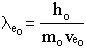11.14
Putting equations 2.22, 2.23 and 11.13 in 11.14, the wavelengthin the moving frame is :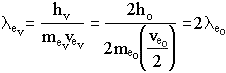11.15
Equation 11.15 shows that the electron wavelength orbiting the moving atom is twice as long as the electron wavelength of the atom at rest. Consequently, the radius of the electron orbit is twice as large, when the atom moves at velocity v=0.866c.  This satisfies perfectly the conditions required above in equation 11.2 when the requirements of quantum mechanics are applied.
This satisfies the wave condition of the constructive interference of the electron wave after each translation since the radius of the orbit (therefore its circumference) of the moving atom is twice as large as the radius for the atom at rest as illustrated on figure 11.1.
Furthermore, when all these fundamental conditions are perfectly satisfied, the frequency of emission of light between electronic transitions is reduced by two, since the energy between the states is reduced by two when the atom is moving, exactly as observed experimentally from the red shift of spectral lines and from the slowdown of moving atomic clocks. It is this absolute reduction of frequency of a moving clock located in a moving frame that has been erroneously interpreted by Einstein as time dilation.
We must then conclude that the predicted absolute change of parameters inside a moving frame, resulting from mass-energy conservation is coherent inside moving atoms. We must also note that all the transformations given above are in perfect agreement with constant absolute electric charges in all frames when kinetic energy is added to atoms. (see equations 11.7 and 11.8). We recall that this absolute electric field is similar to the absolute gravitational field shown in chapters four and five. This agreement proves the invariability of the electric forces as well as the quadratic decrease of the electric field around charges in all frames.  This result agrees perfectly with the well observed experiment showing that electric charges moving at high velocity in a magnetic field travel along a larger radius of curvature corresponding to a different value of e/m. This smaller ratio of electric charge over electron mass is due to an increase of mass (due to kinetic energy) while there is no change of electric charge. Therefore, experimental results show that the ratio of the electric charge over the mass of electrons (e/m) is different in fast moving particles.

11.4 - Sommerfeld Fine Structure.
The prediction of the advance of the perihelion of Mercury seen in chapter five is not the sole example of the success of the principle of mass-energy conservation and classical mechanics. There is also a well documented example in atomic and molecular physics in which it is clearly observed that the principle of mass-energy conservation influences the electronic structure inside atoms. There are many similarities between Mercury moving inside the gravitational field of the Sun and the electrons of atoms orbiting inside the electric potential of the proton. However, an important difference is that the electron mass is not concentrated into a relatively small location with respect to the size of the atom contrary to the case of Mercury and the Sun.
Since electrons exist as waves, the electric potential between the electron cloud and the proton can be calculated using the wave distribution given in quantum mechanics. This leads to the same average energy and distance ao that we would find if all the electron was concentrated at a distance equal to the Bohr radius from the proton. Consequently, one can calculate the potential of that electron cloud using quantum mechanics, as if it were located at a distance from the proton, equal to the Bohr radius. That electron cloud can either oscillate through the proton if the angular momentum is zero or around it, if the angular momentum is not zero.
When the electron cloud is trapped into the electric field of a proton, an hydrogen atom is formed. During its formation, energy is given up as emitted radiation. This is similar to the energy that Mercury must release when it is trapped into the Sun's gravitational potential. The electron cloud can be distributed according to many configurations having different energies corresponding to different quantum states. Consequently, during the formation of each of those states, the electron loses mass the same way Mercury does when it is trapped in the Sun's gravitational potential.
Let us use the Bohr model in which an electron moves on an orbit around the nucleus. We know that the Rydberg states of hydrogen correspond to electrons traveling on an orbit whose circumference is exactly equal to an integer number of the wavelength of the electron. Then, there is a constructive interference of the electron wave when moving to the next orbit around the nucleus. The number of wavelengths forming the orbit is equal to the principal quantum number. This model is compatible with the energies calculated by quantum mechanics.
Experimentally, after the Rydberg states were measured, it was noticed that the transitions between these states are not as simple as originally expected. It was discovered that the transitions between each pair of states are generally made of several very close spectral lines. Sommerfeld carried out calculations using general relativity and he discovered that instead of simple transitions between quantum states, there should be multiple transitions due to the fine structure.
Due to the change of electron mass as a function of its distance from the proton, the wavelength of the electron changes. Consequently, the radius of the orbit changes because it is necessary to have an integer number of wavelengths in a circumference. Due to that change of the distance from the proton, the electrostatic potential changes so that the electron energy becomes different. Consequently, the force (not the field) between the electron and the proton does not follow exactly a quadratic function. Therefore the electron orbit around the proton precesses as in the case of Mercury around the Sun as given in equation 5.52. Due to this precession, the transitions between different quantum states have slightly different energies depending on the relative direction of the velocity of the electron around the nucleus involved in the quantum transition.
Experimentally, the fine structure is well known. The Sommerfeld fine structure constant is equal to:11.16
where h is the Planck parameter.
This fine structure term is observed between all quantum states as long as transitions are allowed by the selection rules. Sommerfeld's fine structure is explained in many textbooks . It is often illustrated by precessing ellipses forming rosettes identical to the path of Mercury on figure 6.2.
The Sommerfeld fine structure constant can be explained more accurately using the principle of mass-energy conservation as done in the case of the orbit of Mercury. However, this is beyond the scope of this book. We will limit our explanations to this qualitative description. We understand now that the fine structure inside atoms is due to the principle of mass-energy conservation. Of course, Sommerfeld's calculations do not lead to a complete agreement in the case of an electron, because one must consider the electron spin. However, this last correction is irrelevant in the case of Mercury.

11.5 - Atomic Structure inside Free Falling Atoms.
Let us study a hydrogen atom falling freely in a gravitational field. We can assume that the atom was initially located in outer space before it slowly started to drift and accelerate gradually toward the Sun. Then, gradually, the hydrogen atom acquires a high velocity. An observer accompanying the falling mass would not feel any internal acceleration. We will now calculate the absolute rate of the falling clock.
Let us examine this problem separating mathematically the two components of energy acting on the falling mass. With respect to a rest frame in outer space, the speeding hydrogen atom is now at a location where there exists a gravitational potential. We have seen that to calculate the exact mass of the particle, this potential must be taken into account. Furthermore, the falling hydrogen has acquired a velocity which must also be taken into account.
We have seen in equation 1.22 that the gravitational potential, where the atom is now located, is such that the mass of the particle has decreased and is now different from its mass in outer space. We also know that the kinetic energy increases the mass of the particle by an amount, which must be equal to the mass lost due to the potential energy.
This can be easily calculated and we see that the decrease of mass due to the gravitational potential compensates exactly the increase of mass due to kinetic energy. Consequently, the absolute mass of the particle (proton and electron) does not change while it is falling.

11.6 - High Potentials and Higher Order Terms.
Contrary to Einstein, in this book we have not arbitrarily postulated that physical quantities are invariant in all frames. We have used only the principle of mass-energy conservation. However, we have found that when we consider the zero and first orders of v/c (or the gravitational potential), the physical laws appear (almost) invariant in all frames as arbitrarily assumed by Einstein. In that particular case, the physical consequences are almost all identical to what Einstein found with his arbitrary postulate. However, our results are obtained using solely the principle of mass-energy conservation. The physical laws derived from the use of the first order of v/c are invariant, up to the point we reach higher order terms in (v/c)2 and other still higher terms (however small) that have been neglected.
One could repeat all the above calculations without neglecting the higher order terms. Then, one could have an exact answer to the problem of extreme energies. We can foresee that if we dealt with physical phenomena in which the higher terms were not negligible (correction due to velocity), the physical laws observed might be slightly different. Within those physical limiting conditions, at high energy, the behavior of matter would not correspond to the description we are used to see in a rest frame and in a frame in which the ratio v/c is not too high.
We have to realize that the experimental conditions that correspond to such high energies are quite common in physics. It is clear that when the nucleus of an atom emits particles having energies of millions of electron volts, the second and third order terms of the potential involved are not negligible. Consequently, we expect that the internal phenomena taking place in the nucleus of atoms at such high potential taking into account the higher order terms lead to physics we are not accustomed to see. It is for that reason that nuclear forces are not familiar to us and to classical mechanics. We believe that the principle of mass-energy conservation is one of the ultimate principles in physics that possesses the wonderful power of informing us, in a logical way, on the correct physical nature of the forces involved in nuclear and particle physics. Mass-energy conservation is relevant everywhere in physics and can be applied everywhere in nature, especially when enormous potentials are involved as in the nucleus of atoms and at the center of the stars.
A general study of physics in which the principle of mass-energy conservation is fully applied is beyond the scope of this book. However, we are convinced that a physical and realistic description of our physical world can be logically achieved without having to involve the non-realistic, the non-conservation of mass-energy and the contradictory hypotheses used in modern physics .

11.7 - References.
 H. Semat, Introduction to Atomic and Nuclear Physics, Holt, Rinehart and Winston, Forth edition, P. 245, 1962.
 P. Marmet, Les Éditions du Nordir, c/o R. Yergeau, 165 Waller Street, Simard Hall, Ottawa, On. Canada K1N 6N5, 144p. 1993.

11.8 - Symbols and Variables.

 ao Bohr radius of the atom at rest av Bohr radius of the moving atom Eo Electric energy of the atom at rest Ev Electric energy of the moving atom lo de Broglie wavelength of the atom at rest lv de Broglie wavelength of the moving atom mo mass of the atom at rest mv mass of the moving atom vo velocity of the electron relative to the proton of the atom at rest vv velocity of the electron relative to the proton of the moving atom

<><><><><><><><><><><><>

Chapter10    Contents      Chapter 12